Categories: ,

# Ohm’s Law Apparatus:

To determine the resistance per cm of a given wire by plotting a graph of potential difference versus current, and hence to determine its resistivity.

According to the Ohm’s law, “The current flowing through a conductor is directly proportional to the potential difference across its ends provided the physical conditions (temperature, dimensions, pressure) of the conductor remains the same.” If I be the current flowing through a conductor and V be the potential difference across its ends, then according to Ohm’s Law,

##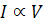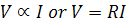where, R is the constant of proportionality. It is known as resistance of the conductor. Or

##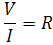..the working formula

R depends upon the material, temperature and dimensions of the conductor.

In S.I. units, the potential difference V is measured in volt and the current I in ampere, the resistance R is measured in ohm.

How do we establish the current-voltage relationship?

To establish the current-voltage relationship, it is to be shown that the ratio V / I remains constant for a given resistance, therefore a graph between the potential difference(V) and the current (I) must be a straight line.

How then do we find the unknown values of resistance?

It is the constant ratio that gives the unknown values of resistance,

##For a wire of uniform cross-section, the resistance depends on the length l and the area of cross-section A. It also depends on the temperature of the conductor. At a given temperature the resistance,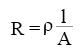where ρ is the specific resistance or resistivity and is characteristic of the material of wire.

Hence, the specific resistance or resistivity of the material of the wire,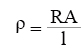If ‘r’ is the radius of the wire, then the cross sectional area, A = πr². Then the specific resistance or resistivity of the material of the wire is,Lerning Outcomes:

• Students learn Ohm’s law.
• Students know the relation between voltage, current and resistance.
• Students learn how to find out the resistivity of the material  of a give wire.

## Reviews

There are no reviews yet.

# Materials Required:

• A resistance wire
• A voltmeter and an ammeter of appropriate range
• A battery (battery eliminator)
• A rheostat
• A metre scale
• One way key
• Connecting wires
• A piece of sand paper
• Screw gauge

# Real Lab Procedure:

• First we'll draw the circuit diagram.
• Arrange the apparatus in the same manner as given in the arrangement diagram.
• Clean the ends of the connecting wires with sand paper to remove insulation, if any.
• Make neat, clean and tight connections according to the circuit diagrams. While making connections ensure that +ve marked terminals of the voltmeter and ammeter are joined towards the +ve terminals of the battery.
• Determine the least count of voltmeter and ammeter, and also note the zero error, if any.
• Insert the key K, slide the rheostat contact and see that the ammeter and voltmeter are working properly.
• Adjust the sliding contact of the rheostat such that a small current passes through the resistance coil or the resistance wire.
• Note down the value of the potential difference V from the voltmeter and current I from the ammeter.
• Shift the rheostat contact slightly so that both the ammeter and voltmeter show full divisions readings and not in fraction.
• Record the readings of the voltmeter and ammeter.
• Take at least six sets of independent observations.
• Record the observations in a tabular column
• Now, cut the resistance wire at the points where it leaves the terminals, stretch it and find its length by the meter scale.
• Then find out the diameter and hence the radius of the wire using the screw gauge and calculate the cross- sectional area A (πr²).
• Plot a graph between current (I) along X-axis and potential difference across the wire (V) along Y-axis.
• The graph should be a stright line.
• Determine the slope of the graph. The slope will give the value of resistance (R) of the material of the wire.
• Calculate the resistance per centimeter of the wire.
• Now,calculate the resistivity of the material of the wire using the formula,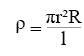# Simulator

• Select the metal form the drop down list.
• Select the length of the wire from the slider.
• Select the diameter of the wire using the slider.
• Select the resistance of the rheostat using the slider.
• To see the circuit diagram, click on the ‘Show circuit diagram’ check box seen inside the simulator window.
• Connections can be made as seen in the circuit diagram by clicking and dragging the mouse form one connecting terminal to the other connecting terminal of the devices to be connected.
• Drag the wire and place it on the voltmeter to have it connected.
• Once all connections are made, click and drag the key to insert it into the switch.
• Slowly move the rheostat contact to change the voltage and current in the voltmeter and ammeter accordingly.
• Calculate the resistivity of the materials based on the length of the wire selected.
• Click on the ‘Show result’ check box to verify your result.
• Click on the ‘Reset’ button to redo the experiment.

# Observations:

1. Length Length of the resistance wire l =......cm
2. Range Range of the given ammeter = .......A. Range of the given voltmeter = .......V.
3. Least count Least count of ammeter = .......A. Least count of voltmeter = .......V.
4. Zero error Zero error in ammeter, e1 = .......A. Zero error in voltmeter,e2 = ......V.
5. Zero correction Zero correction for ammeter, (-e1) = .......A. Zero correction for voltmeter, (-e2) = ......V.
Table for Ammeter and Voltmeter Readings:
 Serial no of obs Ammeter Reading I (A) Voltmeter Reading V (V) (Ohm) Observed Corrected Observed Corrected 1 2 3 4
Mean value of resistance, R = ....................ohms. Results: Resistance per cm of the wire is ........................Ωcm-1. Cross-sectional area of the wire, A = πr² = ...........cm² Resistivity of the material of the wire, ρ = ................Ωcm